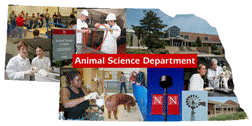Animal Science DepartmentFaculty Papers and Publications in Animal Science

Date of this Version

August 1985

Published in Journal of Dairy Science. Copyright © 1965 American Dairy Science Association. Used by permission.

Abstract

Covariances among first-lactation milk records expressed as deviations from herdmate averages of Holstein cows related as cousins of varying degree, as daughterdan1, as full and maternal sibs, and as aunt-niece of varying degree were examined to determine whether genetic maternal effects are important. The multiple regression of the expected coefficients of additive direct, additive maternal, dominance direct, and dominance maternal genetic variance components; and additive direct by additive maternal and dominance direct by dominance maternal genetic covariances on the 24 estimated covariances between pairs of relatives gave a multiple correlation coefficient of .95. The regression of actual covariance on the coefficient o£ additive direct genetic variance gave a correlation of .92. These results suggest for these data that only additive genetic effects are important for this trait. Additive genetic variance accounted for 38% of total variance. The intercept of the regression line indicated that the average environmental covariance among pairs of relatives accounts for 2 to 3% of the total variance.

COinS